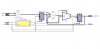# Please help me in VHDL MuX or else my life is in danger

#### erick232

I have to make an exact positioning of this FIR filter. In which Multiplexer should come first then adder.please , please help me

I have attached my Synthesized print screen and the structure which I want to bring it..

Problem is "" Mux should come first then adder "" please help me. I know about me that am not a bad vhdl programmer and I learning from my mistakesas newbie

LIBRARY IEEE;
USE IEEE.std_logic_1164.all;
USE IEEE.std_logic_signed.all;
use IEEE.std_logic_arith.all;
use IEEE.math_real.all;

entity DataPath2 is
port ( f,c:in std_logic_vector(15 downto 0);
clk, reset: in std_logic;
oe : out bit;
resultut std_logic_vector(15 downto 0));
end DataPath2;

architecture Behavioral of DataPath2 is
signal s2,s3: std_logic_vector(15 downto 0):=(others=>'0');
type state_type is(st0,st1,st2,st3,st4,st5);
signal pres_state,next_state :state_type;
signal temp_reg,ts2,ts3 : std_logic_vector(18 downto 0):=(others=>'0');
signal sel :bit;
signal reg : std_logic_vector (31 downto 0):=(others=>'0');
begin

datapathrocess (pres_state,s2,s3,f,c,reg)
begin

s2 <= reg (31 downto 16);
next_state<= pres_state;
oe <= '0'; -- Default value for oe
sel <= '1'; -- Default value for sel
case pres_state is
when st0 =>
sel <= '0'; -- new value for sel
next_state <= st1;
when st1 =>
next_state <= st2;
when st2 =>
next_state <= st3;
when st3 =>
next_state <= st4;
when st4 =>
next_state <= st5;
when st5 =>
oe <='1'; -- new value for oe
next_state <= st0;
end case;
end process;

ts2 <= "000"& s2;
ts3 <= "000"& s3;
temp_reg <= ts2 + ts3;
result <= s3;

process (clk,reset)
begin
if (reset='1') then
pres_state <= st0;
s3 <= (others =>'0');
reg <= (others =>'0');
elsif (rising_edge (clk))then
reg <=f*c;
if sel='0' then
s3 <= s2;
else
s3 <= temp_reg(18 downto 3);
end if;
pres_state <= next_state;
end if;
end process;
end Behavioral;

#### Attachments

• VR_FIR.zip
13.3 KB · Views: 127
•s1.png
19.9 KB · Views: 273

#### joris

You might find it easier to write it as with a single process; it will probably be more straightforward to implement it that way (following the diagram)

#### erick232

I mean , it should be implemented using melay state machine,.! So, have to use two process one is sequential and other one is combinatorial

#### joris

You can look at http://www.xilinx.com/itp/xilinx7/books/data/docs/xst/xst0028_5.html#wp240944 for an example how to implement a state diagram using two processes.

The seperate 'next_process' idiom seems to be more usefull for an implementation using three processes, see http://www.xilinx.com/itp/xilinx7/books/data/docs/xst/xst0028_5.html#wp241028

Don't implement part of the combinatorial code outside of a process, it's not very clear what you're doing.

For intermediate results (which you need to read after writing them), use variables instead of signals; For example, the output of the "mux" should be written in a variable as you will feed it into the adder afterwards;

Last edited: